### MEMO 2016 ekipno problem 8

Kvaliteta:
Avg: 0.0
Težina:
Avg: 6.0

We consider the equation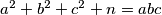$a^2 + b^2 + c^2 + n = abc$, where$a$,$b$,$c$ are positive integers.

Prove:

(a) There are no solutions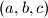$(a, b, c)$ for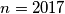$n = 2017$.

(b) For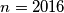$n = 2016$,$a$ must be divisible by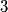$3$ for every solution$(a, b, c)$.

(c) The equation has infinitely many solutions$(a, b, c)$ for$n = 2016$.

Source: Srednjoeuropska matematička olimpijada 2016, ekipno natjecanje, problem 8